# 十四、对象识别

• 对象检测和对象识别有什么区别
• 什么是密集特征检测器
• 什么是视觉词典
• 如何建立特征向量
• 什么是监督和无监督学习
• 什么是支持向量机以及如何使用它们来构建分类器
• 如何识别未知图像中的对象

# 对象检测与对象识别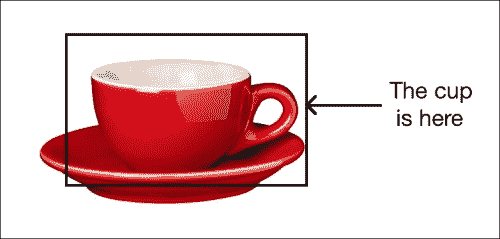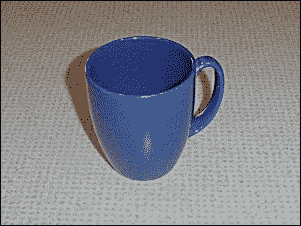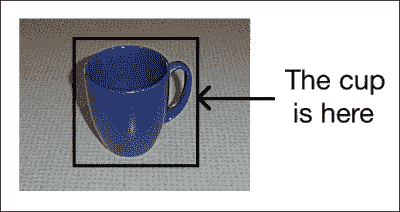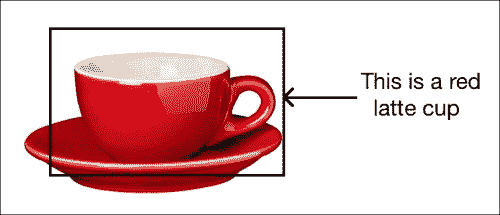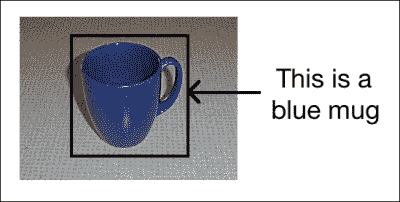# 什么是密集特征检测器？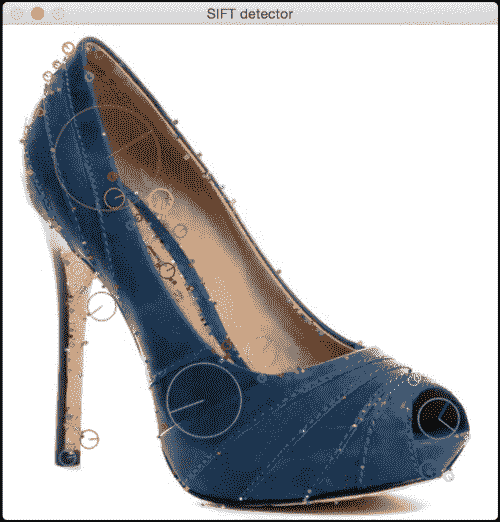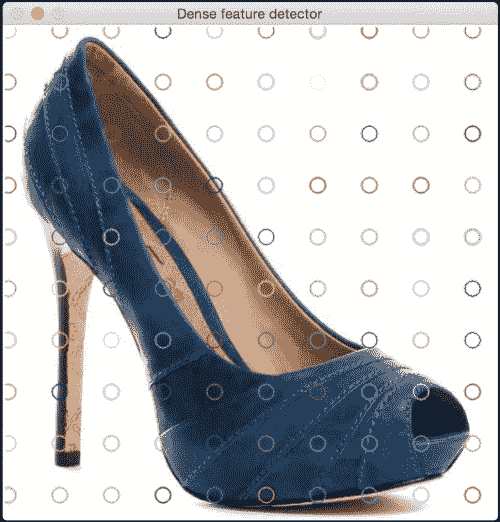import cv2
import numpy as np

class DenseDetector(object):
def __init__(self, step_size=20, feature_scale=40, img_bound=20):
# Create a dense feature detector
self.detector = cv2.FeatureDetector_create("Dense")

# Initialize it with all the required parameters
self.detector.setInt("initXyStep", step_size)
self.detector.setInt("initFeatureScale", feature_scale)
self.detector.setInt("initImgBound", img_bound)

def detect(self, img):
# Run feature detector on the input image
return self.detector.detect(img)

if __name__=='__main__':
input_image_sift = np.copy(input_image)

# Convert to grayscale
gray_image = cv2.cvtColor(input_image, cv2.COLOR_BGR2GRAY)

keypoints = DenseDetector(20,20,5).detect(input_image)

# Draw keypoints on top of the input image
input_image = cv2.drawKeypoints(input_image, keypoints,
flags=cv2.DRAW_MATCHES_FLAGS_DRAW_RICH_KEYPOINTS)

# Display the output image
cv2.imshow('Dense feature detector', input_image)

# Initialize SIFT object
sift = cv2.SIFT()

# Detect keypoints using SIFT
keypoints = sift.detect(gray_image, None)

# Draw SIFT keypoints on the input image
input_image_sift = cv2.drawKeypoints(input_image_sift,
keypoints, flags=cv2.DRAW_MATCHES_FLAGS_DRAW_RICH_KEYPOINTS)

# Display the output image
cv2.imshow('SIFT detector', input_image_sift)

# Wait until user presses a key
cv2.waitKey()


# 什么是视觉词典？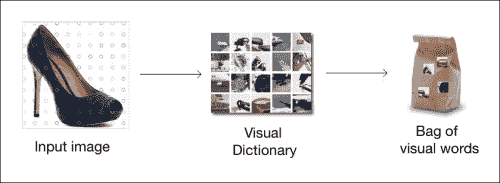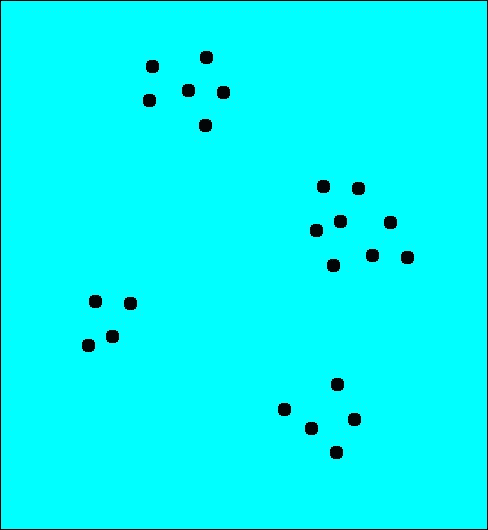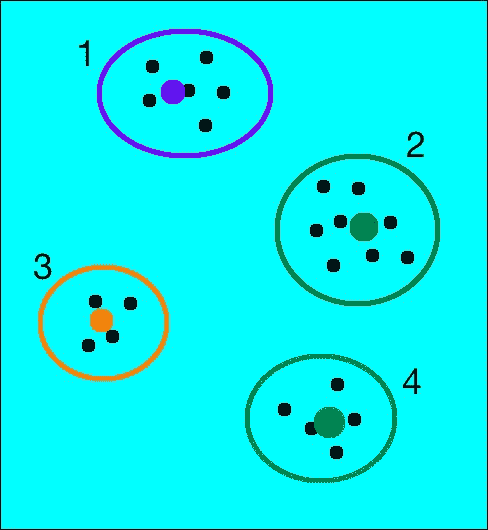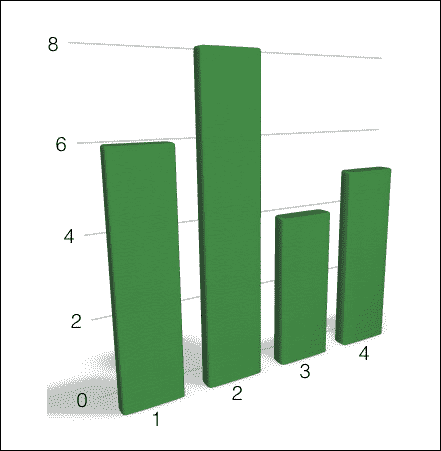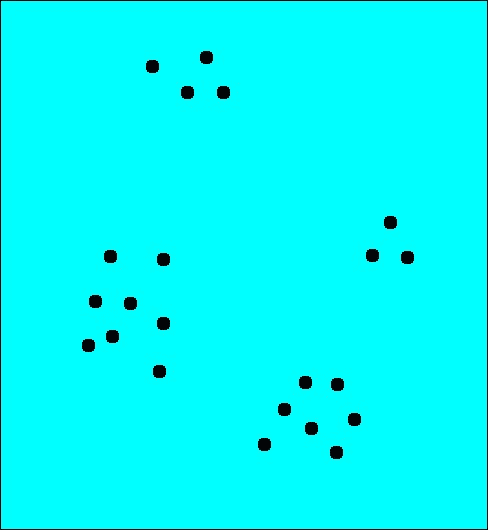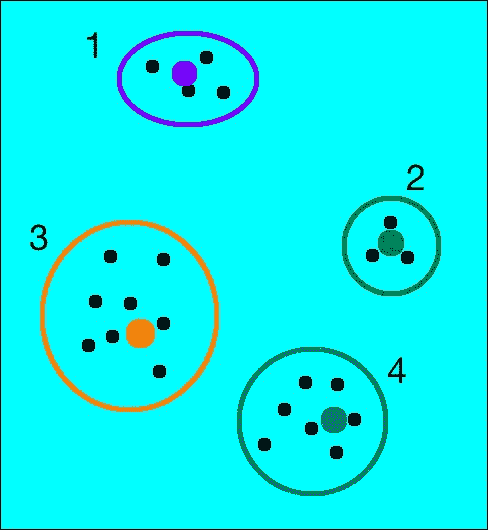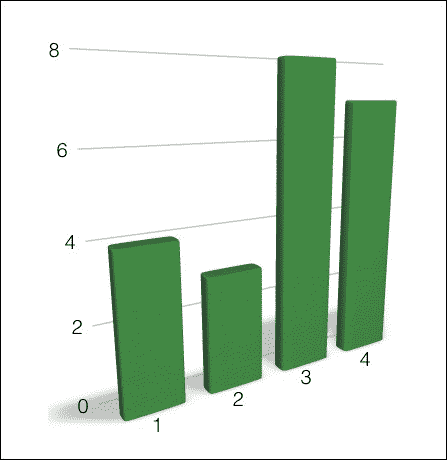# 什么是支持向量机？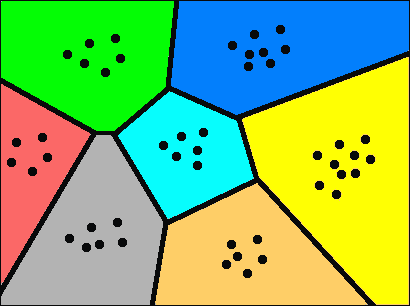## 如果我们不能用简单的直线分离数据怎么办？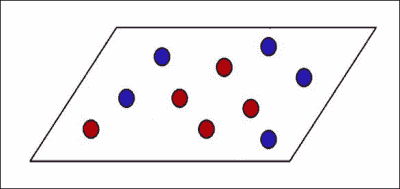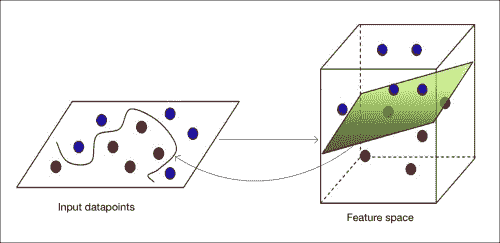SVM 的主题确实很深，我们将无法在此处进行详细讨论。 如果您真的有兴趣，可以在线找到大量材料。 您可以通过简单的教程来更好地理解它。

# 我们如何实际实现呢？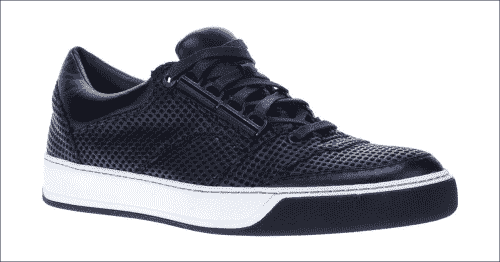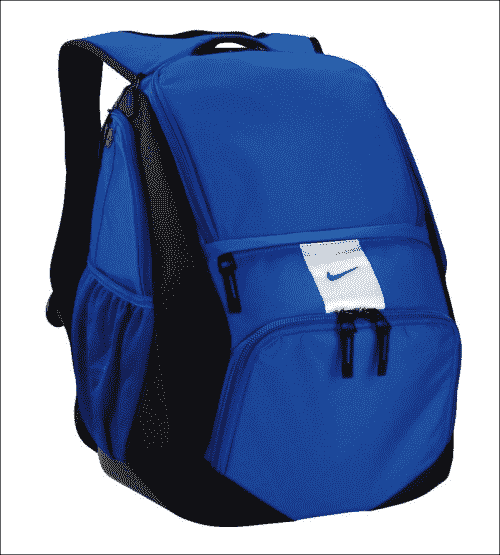import os
import sys
import argparse
import cPickle as pickle
import json

import cv2
import numpy as np
from sklearn.cluster import KMeans

def build_arg_parser():
parser = argparse.ArgumentParser(description='Creates features for given images')
required=True, help="Folders containing the training images. \
The first element needs to be the class label.")
help="Base file name to store the codebook")
help="Base file name to store the feature map")

return parser

combined_data = []

if not os.path.isdir(input_folder):
raise IOError("The folder " + input_folder + " doesn't exist")

# Parse the input folder and assign the  labels
for root, dirs, files in os.walk(input_folder):
for filename in (x for x in files if x.endswith('.jpg')):
combined_data.append({'label': label, 'image': os.path.join(root, filename)})

return combined_data

class FeatureExtractor(object):
def extract_image_features(self, img):
# Dense feature detector
kps = DenseDetector().detect(img)

# SIFT feature extractor
kps, fvs = SIFTExtractor().compute(img, kps)

return fvs

# Extract the centroids from the feature points
def get_centroids(self, input_map, num_samples_to_fit=10):
kps_all = []

count = 0
cur_label = ''
for item in input_map:
if count >= num_samples_to_fit:
if cur_label != item['label']:
count = 0
else:
continue

count += 1

if count == num_samples_to_fit:
print "Built centroids for", item['label']

cur_label = item['label']
img = resize_to_size(img, 150)

num_dims = 128
fvs = self.extract_image_features(img)
kps_all.extend(fvs)

kmeans, centroids = Quantizer().quantize(kps_all)
return kmeans, centroids

def get_feature_vector(self, img, kmeans, centroids):
return Quantizer().get_feature_vector(img, kmeans, centroids)

def extract_feature_map(input_map, kmeans, centroids):
feature_map = []

for item in input_map:
temp_dict = {}
temp_dict['label'] = item['label']

print "Extracting features for", item['image']
img = resize_to_size(img, 150)

temp_dict['feature_vector'] = FeatureExtractor().get_feature_vector(
img, kmeans, centroids)

if temp_dict['feature_vector'] is not None:
feature_map.append(temp_dict)

return feature_map

# Vector quantization
class Quantizer(object):
def __init__(self, num_clusters=32):
self.num_dims = 128
self.extractor = SIFTExtractor()
self.num_clusters = num_clusters
self.num_retries = 10

def quantize(self, datapoints):
# Create KMeans object
kmeans = KMeans(self.num_clusters,
n_init=max(self.num_retries, 1),
max_iter=10, tol=1.0)

# Run KMeans on the datapoints
res = kmeans.fit(datapoints)

# Extract the centroids of those clusters
centroids = res.cluster_centers_

return kmeans, centroids

def normalize(self, input_data):
sum_input = np.sum(input_data)
if sum_input > 0:
return input_data / sum_input
else:
return input_data

# Extract feature vector from the image
def get_feature_vector(self, img, kmeans, centroids):
kps = DenseDetector().detect(img)
kps, fvs = self.extractor.compute(img, kps)
labels = kmeans.predict(fvs)
fv = np.zeros(self.num_clusters)

for i, item in enumerate(fvs):
fv[labels[i]] += 1

fv_image = np.reshape(fv, ((1, fv.shape)))
return self.normalize(fv_image)

class DenseDetector(object):
def __init__(self, step_size=20, feature_scale=40, img_bound=20):
self.detector = cv2.FeatureDetector_create("Dense")
self.detector.setInt("initXyStep", step_size)
self.detector.setInt("initFeatureScale", feature_scale)
self.detector.setInt("initImgBound", img_bound)

def detect(self, img):
return self.detector.detect(img)

class SIFTExtractor(object):
def compute(self, image, kps):
if image is None:
print "Not a valid image"
raise TypeError

gray_image = cv2.cvtColor(image, cv2.COLOR_BGR2GRAY)
kps, des = cv2.SIFT().compute(gray_image, kps)
return kps, des

# Resize the shorter dimension to 'new_size'
# while maintaining the aspect ratio
def resize_to_size(input_image, new_size=150):
h, w = input_image.shape, input_image.shape
ds_factor = new_size / float(h)

if w < h:
ds_factor = new_size / float(w)

new_size = (int(w * ds_factor), int(h * ds_factor))
return cv2.resize(input_image, new_size)

if __name__=='__main__':
args = build_arg_parser().parse_args()

input_map = []
for cls in args.cls:

assert len(cls) >= 2, "Format for classes is <label> file"
label = cls

# Building the codebook
print "===== Building codebook ====="
kmeans, centroids = FeatureExtractor().get_centroids(input_map)
if args.codebook_file:
with open(args.codebook_file, 'w') as f:
pickle.dump((kmeans, centroids), f)

# Input data and labels
print "===== Building feature map ====="
feature_map = extract_feature_map(input_map, kmeans, centroids)
if args.feature_map_file:
with open(args.feature_map_file, 'w') as f:
pickle.dump(feature_map, f)


## 代码内部发生了什么？

Quantizer类旨在实现向量量化并构建特征向量。 对于从图像中提取的每个关键点，get_feature_vector方法会在我们的词典中找到最接近的视觉单词。 通过这样做，我们最终基于可视词典构建了直方图。 现在，将每个图像表示为一组视觉单词的组合。 因此，名称为词袋

import os
import sys
import argparse

import cPickle as pickle
import numpy as np
from sklearn.multiclass import OneVsOneClassifier
from sklearn.svm import LinearSVC
from sklearn import preprocessing

def build_arg_parser():
parser = argparse.ArgumentParser(description='Trains the classifier models')
help="Input pickle file containing the feature map")
help="Output file where the pickled SVM model will be stored")
return parser

# To train the classifier
class ClassifierTrainer(object):
def __init__(self, X, label_words):
# Encoding the labels (words to numbers)
self.le = preprocessing.LabelEncoder()

# Initialize One vs One Classifier using a linear kernel
self.clf = OneVsOneClassifier(LinearSVC(random_state=0))

y = self._encodeLabels(label_words)
X = np.asarray(X)
self.clf.fit(X, y)

# Predict the output class for the input datapoint
def _fit(self, X):
X = np.asarray(X)
return self.clf.predict(X)

# Encode the labels (convert words to numbers)
def _encodeLabels(self, labels_words):
self.le.fit(labels_words)
return np.array(self.le.transform(labels_words), dtype=np.float32)

# Classify the input datapoint
def classify(self, X):
labels_nums = self._fit(X)
labels_words = self.le.inverse_transform([int(x) for x in labels_nums])
return labels_words

if __name__=='__main__':
args = build_arg_parser().parse_args()
feature_map_file = args.feature_map_file
svm_file = args.svm_file

with open(feature_map_file, 'r') as f:

# Extract feature vectors and the labels
labels_words = [x['label'] for x in feature_map]

# Here, 0 refers to the first element in the
# feature_map, and 1 refers to the second
# element in the shape vector of that element
# (which gives us the size)
dim_size = feature_map['feature_vector'].shape

X = [np.reshape(x['feature_vector'], (dim_size,)) for x in feature_map]

# Train the SVM
svm = ClassifierTrainer(X, labels_words)
if args.svm_file:
with open(args.svm_file, 'w') as f:
pickle.dump(svm, f)


## 我们如何构建训练器？

$pip install scikit-learn  我们从标记的数据开始，并将其提供给OneVsOneClassifier方法。 我们有一个classify方法，该方法可以对输入图像进行分类并为其关联标签。 让我们试一下吧？ 确保有一个名为images的文件夹，其中有这三个类的训练图像。 创建一个名为models的文件夹，该文件夹将存储学习模型。 在终端上运行以下命令以创建特征并训练分类器： $ python create_features.py --samples bag img/bag/ --samples dress img/dress/ --samples footwear img/footwear/ --codebook-file models/codebook.pkl --feature-map-file models/feature_map.pkl
$python training.py --feature-map-file models/feature_map.pkl --svm-file models/svm.pkl  现在已经对分类器进行了训练，我们只需要一个模块即可对输入图像进行分类并检测其中的物体。 这是执行此操作的代码： import os import sys import argparse import cPickle as pickle import cv2 import numpy as np import create_features as cf from training import ClassifierTrainer def build_arg_parser(): parser = argparse.ArgumentParser(description='Extracts features \ from each line and classifies the data') parser.add_argument("--input-image", dest="input_image", required=True, help="Input image to be classified") parser.add_argument("--svm-file", dest="svm_file", required=True, help="File containing the trained SVM model") parser.add_argument("--codebook-file", dest="codebook_file", required=True, help="File containing the codebook") return parser # Classifying an image class ImageClassifier(object): def __init__(self, svm_file, codebook_file): # Load the SVM classifier with open(svm_file, 'r') as f: self.svm = pickle.load(f) # Load the codebook with open(codebook_file, 'r') as f: self.kmeans, self.centroids = pickle.load(f) # Method to get the output image tag def getImageTag(self, img): # Resize the input image img = cf.resize_to_size(img) # Extract the feature vector feature_vector = cf.FeatureExtractor().get_feature_vector(img, self.kmeans, self.centroids) # Classify the feature vector and get the output tag image_tag = self.svm.classify(feature_vector) return image_tag if __name__=='__main__': args = build_arg_parser().parse_args() svm_file = args.svm_file codebook_file = args.codebook_file input_image = cv2.imread(args.input_image) print "Output class:", ImageClassifier(svm_file, codebook_file).getImageTag(input_image)  我们都准备好了！ 我们只是从输入图像中提取feature向量，并将其用作分类器的输入参数。 让我们继续看看是否可行。 从互联网上下载随机的鞋类图片，并确保其背景干净。 通过用正确的文件名替换new_image.jpg，运行以下命令： $ python classify_data.py --input-image new_image.jpg --svm-file models/svm.pkl --codebook-file models/codebook.pkl